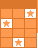Challenge Board: Score points by answering questions correctly.# Solving radical equations - (copy)

Use algebra to solve each radical equation.
1. Get the radical alone in his home
2. Square both sides of the equation (be careful this might create extra answers) (If you squared a binomial don't forget that you will have to write out the binomial twice and distribute)
3. Solve for xThis activity was created by a Quia Web subscriber. Learn more about QuiaCreate your own activities